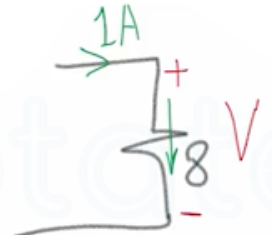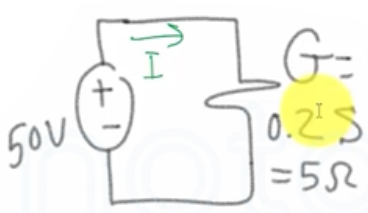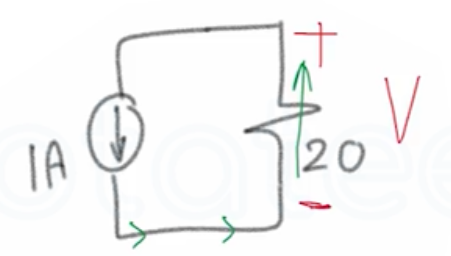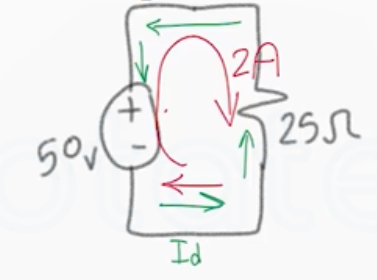Need Help?

Subscribe to Circuit

###### \${selected_topic_name}
• Notes

$\begin{array}{l}{\text { In each circuit following Figures, either the value of } v \text { or } i \text { is not known. }} \\ {\text { a) Calculate the values of } v \text { and } i .} \\ {\text { b) Determine the power dissipated in each resistor. }}\end{array}$$V=I R=1 * 8=8 V$

$P=I^{2} R=(1)^{2} * 8=8 w$

$P=\frac{U^{2}}{R}=\frac{(8)^2}{8}=8 w$

$P=VI=8 * 1=8 w$$R=\frac{1}{G}=\frac{1}{0.2}=5 \Omega$

$V=I R=I * 5$

$50=5 I$

$I=\frac{50}{5}=10 A$

$P=V I=50 * 10=500 \mathrm{W}$

$P=I^{2} R=(10)^{2} * 5=500 l$$V=-I R$

$V=-1 * 20=-20 v$

$P=I^{2} R=(1)^{2}*20=20 \mathrm{w}$$V=-I R=-I*25$

$50=-I * 25$

$I=\frac{-50}{25}=- 2 A$

$P=I^2 R=(2)^{2}*25=100 \mathrm{w}$